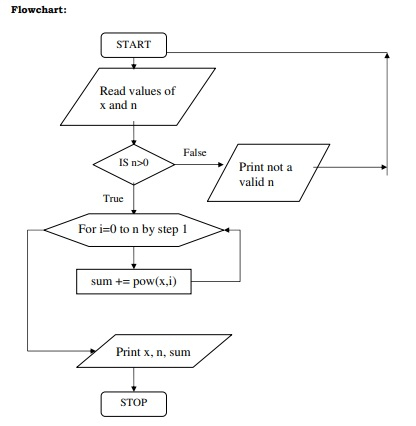# C program to compute geometric progression

## Problem

Write a program to read two numbers, x and n, and then compute the sum of the geometric progression.

1+x+x2+x3+x4+……….+xn

And then, print x,n and sum.

## Solution

The solution to compute the geometric progression in C programming language is given below −

## Algorithm

Refer an algorithm to compute the geometric progression.

Step 1 − Start

Step 2 − Repeat

Step 3 − Read values for x and n at runtime

Step 4 − If n > 0 then

Step 4.1: for i = 0 to n do

Step 4.1.1: sum = sum +pow(x,i)

Step 4.1.2: i = i+1

Step 4.2: print x, n and sum

Step 5 − Else

Step 5.1: print not a valid n value

Step 5.2: goto repeat (junp to step 2)

Step 6 − End if

Step 7 − Stop

## Flowchart

Given below is a flowchart for an algorithm to compute the geometric progression −## Program

Following is the C program to compute the geometric progression

#include <stdio.h>
#include <conio.h>
#include <math.h>
main(){
int x,n,sum=0,i;
start:
printf("enter the values for x and n:");
scanf("%d%d",&x,&n);
if(n>0){
for(i=0;i<=n;i++){
sum = sum+pow(x,i);
}
printf("The sum of the geometric progression is:%d",sum);
}
else{
printf("not a valid n:%d value",n);
getch();
goto start;
}
}

## Output

When the above program is executed, it produces the following result −

enter the values for x and n:4 5
The sum of the geometric progression is:1365